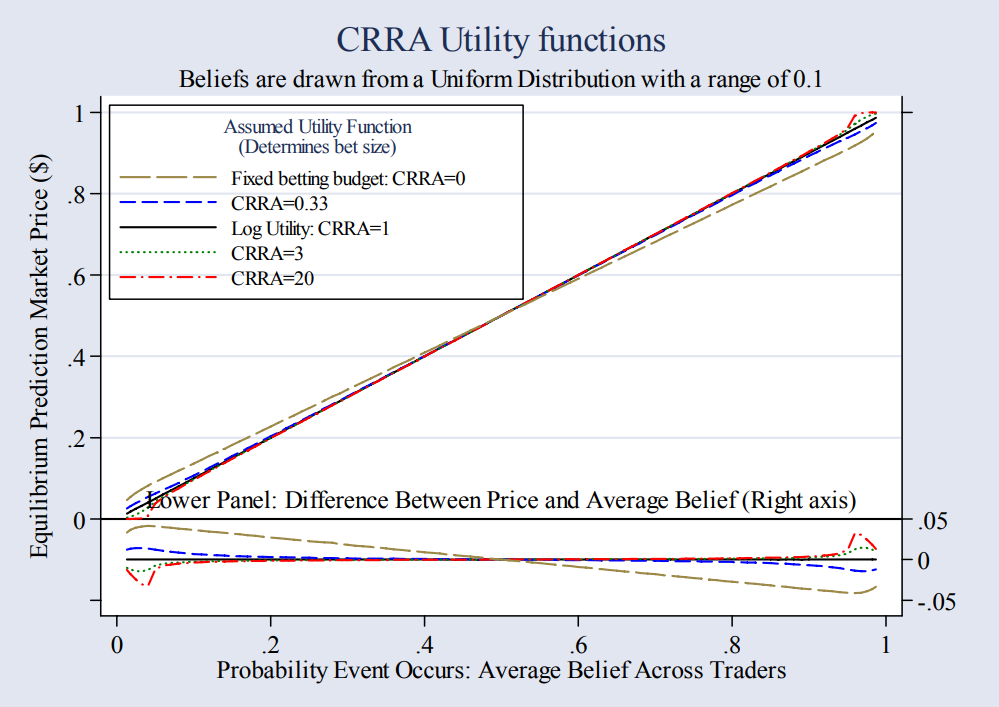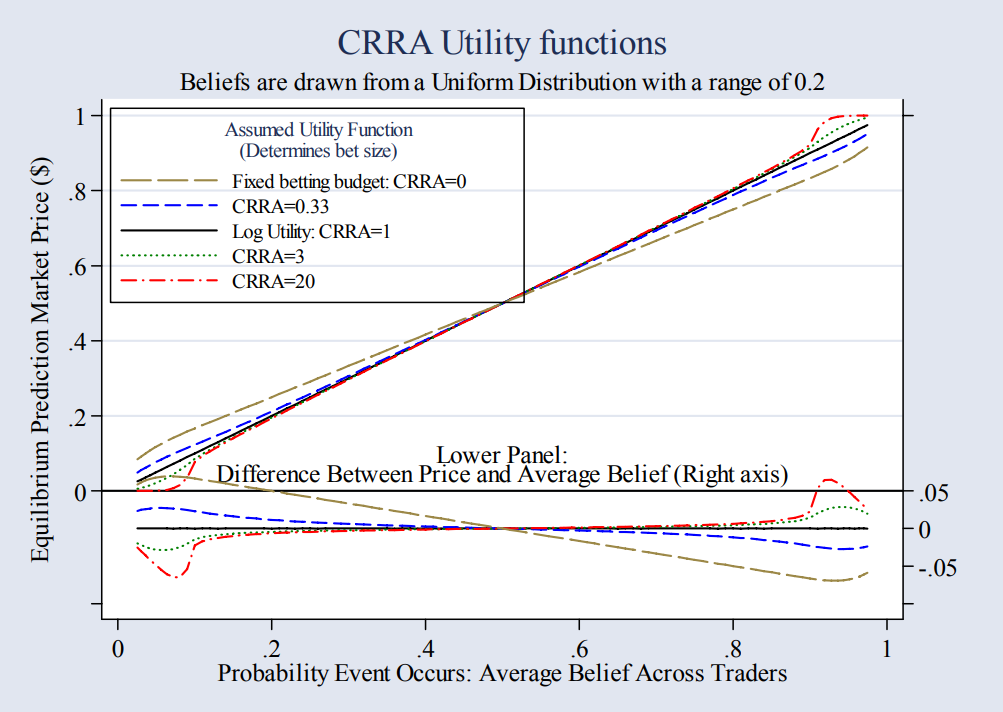We consider a simple prediction market in which traders buy and sell an all-or nothing contract (a binary option) paying \$1 if a specific event occurs, and nothing otherwise. There is heterogeneity in beliefs among the trading population, and following Manski’s notation, we denote trader $j$’s belief that the event will occur as $q_j$. These beliefs are orthogonal to wealth levels $(y)$, and are drawn from a distribution, $F(q)$. Individuals are price-takers and trade so as to maximize their subjectively expected utility. Wealth is only affected by the event via the prediction market, so there is no hedging motive for trading the contract. We first consider the case where traders have log utility, and we endogenously derive their trading activity, given the price of the contract is $\pi$. […] Thus, in this simple model, market prices are equal to the mean belief among traders.

[…]

We now turn to relaxing some of our assumptions. To preview, relaxing the assumption that budgets are orthogonal to beliefs yields the intuitively plausible result that prediction market prices are a wealth-weighted average of beliefs among market traders. And second, the result that the equilibrium price is exactly equal to the (weighted) mean of beliefs reflects the fact that demand for the prediction security is linear in beliefs, which is itself a byproduct of assuming log utility. Calibrating alternative utility functions, we find that prices can systematically diverge from mean beliefs, but that this divergence is typically small. […] The extent of the deviation depends crucially on how widely dispersed beliefs are.

[…]

We start by assuming that beliefs are drawn from a uniform distribution with a range of 10 percentage points, and solve for the mapping between mean beliefs and prices implied by each of the utility functions shown in Figure 1. (We rescale beliefs outside the (0,1) range to 0 or 1.) Figure 2 shows that for moderately dispersed beliefs, prediction market prices tend to coincide fairly closely with the mean beliefs. While there is some divergence, it is typically within a percentage point, although the risk neutral model yields larger differences. […]Figure 2

Figure 3 shows the mapping from prices to probabilities when beliefs are more disperse (in this case the standard deviation and range were doubled). As the dispersion of beliefs widens, the number of traders with extreme beliefs increases, and hence the non-linear response to the divergence between beliefs and prices is increasingly important. As such, the biases evident in Figure 2 become even more evident as the distribution of beliefs widens. Even so, for utility functions with standard levels of risk aversion, these biases are small.Figure 3

Justin Wolfers and Eric Zitzewitz, Interpreting Prediction Market Prices as Probabilities, NBER Working Paper No. 12200, May 2006

Added to diary 20 January 2018